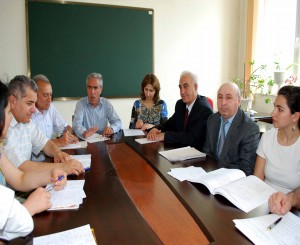﻿ BAKU STATE UNIVERSITY

## The chair of Mathematical methods of control theoryThe chair of Mathematical methods of control theory was established in 1990. Professor S.S.Akhiyev was the first head of the chair.

At present, the chair is functioning in the following content: d.p.-m.s., prof. M.A.Yagubov (head of the chair), d.p.-m.s., prof. H.F.Guliyev, d.p.-m.s., prof. M.A.Sadigov, c.p.-m.s., assoc.prof. T.G.Huseynov, c.p.-m.s., assoc. prof. Sh.Sh.Yusubov, c.p.-m.s., assoc. J.J.Mammadova, c.p.-m.s., assist. A.T.Huseynova, head research assistant L.B.Babayeva.

The following subjects are taught for undergraduate bachelors: variational calculus and optimization methods, special courses, and for master degree: the problem of optimal control in processes described with equations in partial derivatives, theory of controllability and observation, method of dynamic programming, elements of game theory, approximate methods of optimal control theory, stochastic problems of control, extremal problems solution existence, optimal control problems in singular perturbed systems, existence and approximate construction of optimal control.

Over than 200 scientific papers and two textbooks more published by the chaff the chair.

About 30 candidate thesis have been defended under the supervision of prof.S.S.Akhiyev, prof. M.A.Yagubov and prof. H.F.Guliyev.

At the chair scientific-research work on controllability and optimization in processes described with equations in partial derivatives.

Employments:

Yagubov M.H. - Chief of the chair, professor, doctor of physical and mathematical sciences

Guliyev H.F.- professor, doctor of physical and mathematical sciences

Sadigov M.- professor, doctor of physical and mathematical sciences

Huseynov T.H. - associate professor, candidate of physical and mathematical sciences

Yusubov Sh.Sh. - associate professor, candidate of physical and mathematical sciences

Mamedova J.J.- associate professor, candidate of physical and mathematical sciences,

Huseynova A.T.- assistant, candidate of physical and mathematical sciences